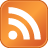## SUPPORT THE SITE WITH A CLICK

Subscribe Rss:## Friday, March 18, 2011

### Ruby Challenge : Prime Numbers - Part I

One of the most important and beautiful fields of mathematics is number theory - the study of numbers and their properties.Despite the fact that mathematicians have been studying numbers for as long as humans have been able to count, the field of number theory is far from being outdated; some of the most exciting and important problems in mathematics today have to do with the study of numbers. In particular, prime numbers are of great interest.

Definition:
A prime number (or a prime) is a natural number that has exactly two distinct natural number divisors: 1 and itself

Basically the purpose of this post is to write the programs for mathematical algorithms.Prime number was most used in math challenges and in technical discussions.

I have just started working on Unit test, algorithms and patterns, so i decided to write test cases for the most of my ruby programs.

Is Prime or Not?
:
```class Prim
def is_prime(n)
return false if n<2
2.upto(Math.sqrt(n).to_i){|i|  return false if n%i==0}
return true
end
end
```